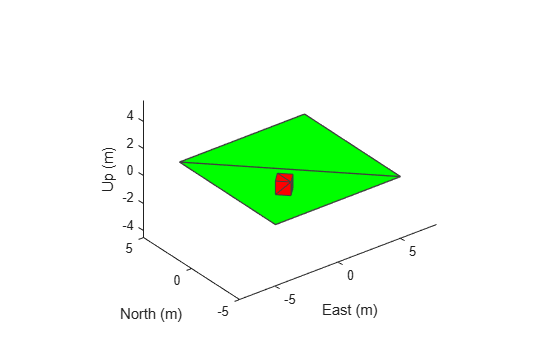Define new inertial frame in robot scenario

## Syntax

``addInertialFrame(scenario,base,name,position,orientation)``
``addInertialFrame(scenario,base,name,tform)``

## Description

````addInertialFrame(scenario,base,name,position,orientation)` adds a new inertial frame to the robot scenario by specifying the base, name, position, and orientation of the new inertial frame.```

example

````addInertialFrame(scenario,base,name,tform)` adds a new inertial frame to the robot scenario by specifying the base, name, and transformation matrix of the new inertial frame.```

## Examples

collapse all

Create a robot scenario. By default, the inertial frames are the ENU and the NED frames.

`scenario = robotScenario`
```scenario = robotScenario with properties: UpdateRate: 10 StopTime: Inf HistoryBufferSize: 100 ReferenceLocation: [0 0 0] MaxNumFrames: 50 CurrentTime: 0 IsRunning: 0 TransformTree: [1x1 transformTree] InertialFrames: ["ENU" "NED"] Meshes: {} Platforms: [0x0 robotPlatform] ```

Add a new inertial frame named `robot` to the scenario.

`addInertialFrame(scenario,"ENU","robot",eul2tform([pi/4 0 0]))`

You can now use the `robot` frame as a reference frame to define other objects in the scenario.

`scenario.InertialFrames`
```ans = 1x3 string "ENU" "NED" "robot" ```

Add plane and box mesh with reference frame in the scenario.

```addMesh(scenario,"Plane",Size=[10 10],Color=[0 1 0]) addMesh(scenario,"Box",Position=[-2 -2 0.5],ReferenceFrame="robot")```

Visualize the scenario.

`show3D(scenario);`## Input Arguments

collapse all

Robot scenario, specified as a `robotScenario` object.

Base of the new inertial frame, specified as a string scalar. The base frame must be defined in the scenario in advance.

Example: `"ENU"`

Data Types: `char` | `string`

Name of the new inertial frame, specified as a string scalar.

Example: `"newFrame"`

Data Types: `char` | `string`

Position of the new inertial frame with respect to the base frame (specified in the `base` argument), specified as a 1-by-3 vector in meters.

Data Types: `single` | `double`

Orientation of the new inertial frame with respect to the base frame (specified in the `base` argument), specified as a `quaternion` object or a 1-by-4 quaternion vector. The specified orientation is from the base frame to the new inertial frame.

Data Types: `single` | `double`

Transformation matrix that maps points in the new frame (specified in the `base` argument) to the base frame, specified as a 4-by-4 homogeneous transform matrix that maps points in the base frame to the new inertial frame.

Example: `[0 0 1 0; 0 1 0 0; -1 0 0 0; 0 0 0 1]`

Data Types: `single` | `double`

## Version History

Introduced in R2022a